Hey, Can someone help me solve 2x²=63-5x using the quadratic formula. ( In algebra 1 by the way)

Question

Hey, Can someone help me solve 2x²=63-5x using the quadratic formula. ( In algebra 1 by the way)

in progress 0
5 months 2021-08-17T02:06:12+00:00 2 Answers 2 views 0

1. Answer (if we’re solving for x):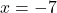orStep-by-step explanation:

1. Rearrange the terms from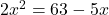to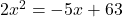2. Move the terms to the left side: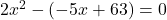3. Use the “sum-product” pattern: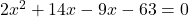4. Get the common factor drom the 2 pairs: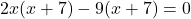5. Rewrite the equation in factored form as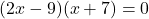6. Create 2 equations from: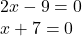7. This means that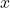can either be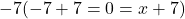or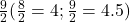2. I don’t want to sound d u m b but use photomath it really helped me with quadratic formula it shows steps too.